# Engineering Electromagnetics W H Hayt Jr and J

• Slides: 72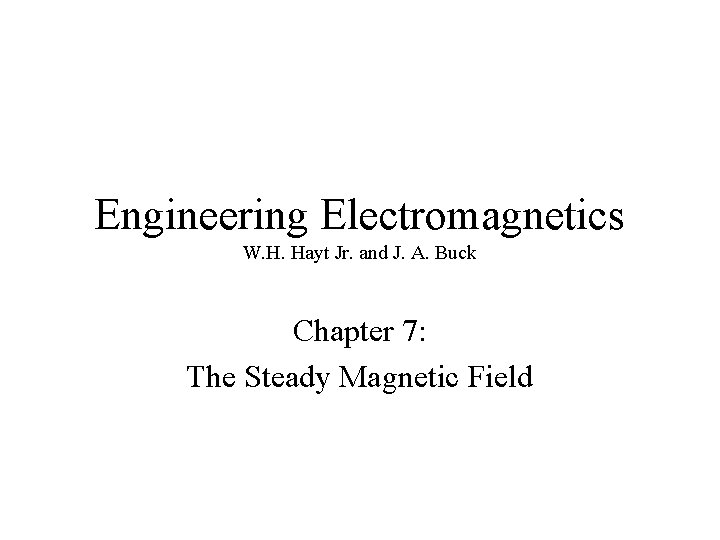Engineering Electromagnetics W. H. Hayt Jr. and J. A. Buck Chapter 7: The Steady Magnetic FieldMotivating the Magnetic Field Concept: Forces Between Currents Magnetic forces arise whenever we have charges in motion. Forces between current-carrying wires present familiar examples that we can use to determine what a magnetic force field should look like: Here are the easily-observed facts: How can we describe a force field around wire 1 that can be used to determine the force on wire 2?Magnetic Field The geometry of the magnetic field is set up to correctly model forces between currents that allow for any relative orientation. The magnetic field intensity, H, circulates around its source, I 1, in a direction most easily determined by the right-hand rule: Right thumb in the direction of the current, fingers curl in the direction of H Note that in the third case (perpendicular currents), I 2 is in the same direction as H, so that their cross product (and the resulting force) is zero. The actual force computation involves a different field quantity, B, which is related to H through B = H in free space. This will be taken up in a later lecture. Our immediate concern is how to find H from any given current distribution.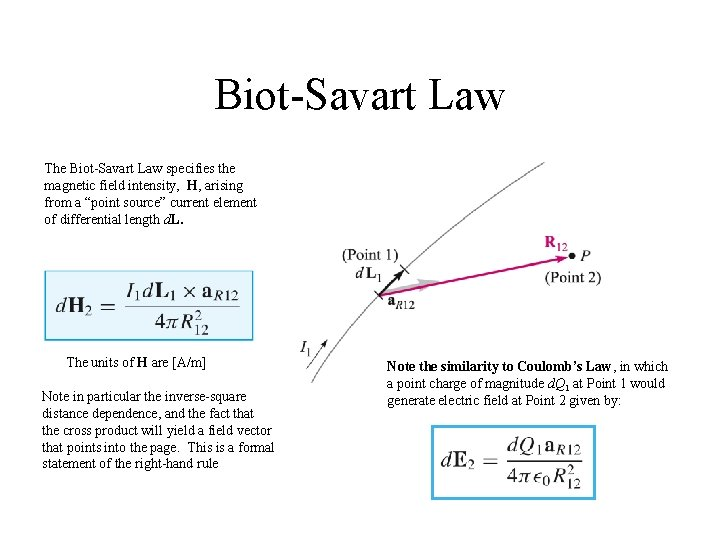Biot-Savart Law The Biot-Savart Law specifies the magnetic field intensity, H, arising from a “point source” current element of differential length d. L. The units of H are [A/m] Note in particular the inverse-square distance dependence, and the fact that the cross product will yield a field vector that points into the page. This is a formal statement of the right-hand rule Note the similarity to Coulomb’s Law, in which a point charge of magnitude d. Q 1 at Point 1 would generate electric field at Point 2 given by:Magnetic Field Arising From a Circulating Current At point P, the magnetic field associated with the differential current element Id. L is To determine the total field arising from the closed circuit path, we sum the contributions from the current elements that make up the entire loop, or The contribution to the field at P from any portion of the current will be just the above integral evalated over just that portion.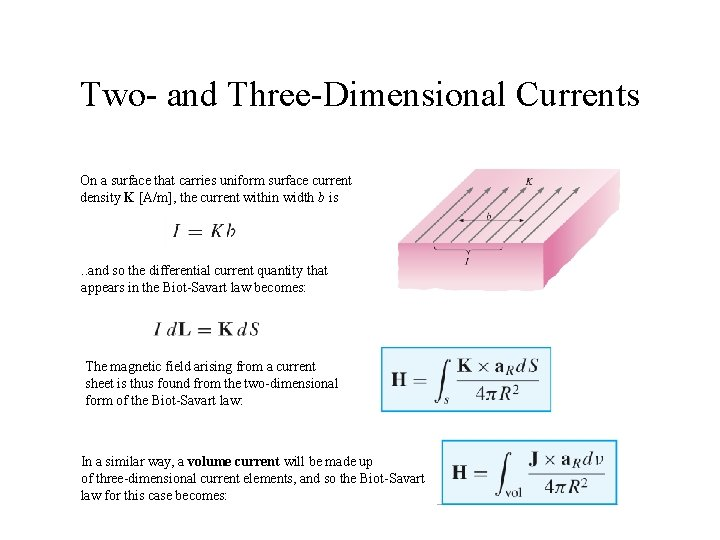Two- and Three-Dimensional Currents On a surface that carries uniform surface current density K [A/m], the current within width b is . . and so the differential current quantity that appears in the Biot-Savart law becomes: The magnetic field arising from a current sheet is thus found from the two-dimensional form of the Biot-Savart law: In a similar way, a volume current will be made up of three-dimensional current elements, and so the Biot-Savart law for this case becomes:Example of the Biot-Savart Law In this example, we evaluate the magnetic field intensity on the y axis (equivalently in the xy plane) arising from a filament current of infinite length in on the z axis. Using the drawing, we identify: and so. . so that: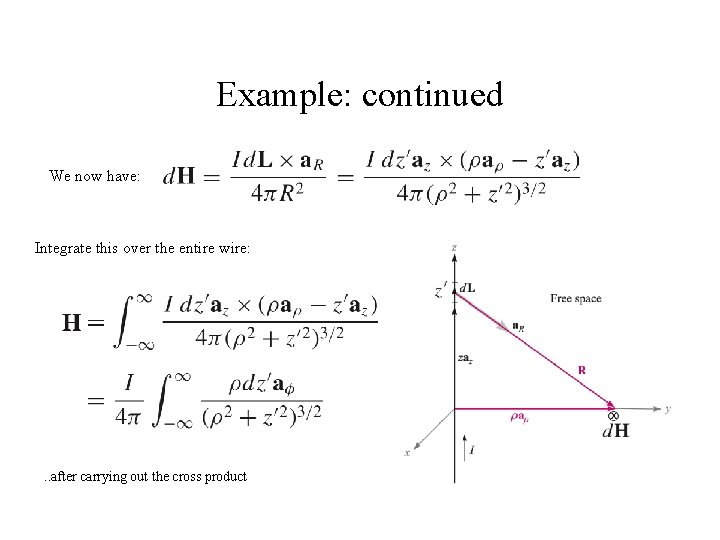Example: continued We now have: Integrate this over the entire wire: . . after carrying out the cross product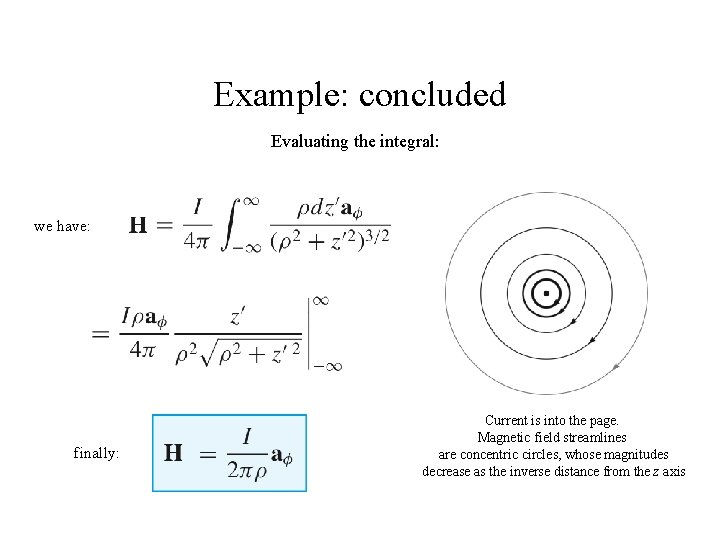Example: concluded Evaluating the integral: we have: finally: Current is into the page. Magnetic field streamlines are concentric circles, whose magnitudes decrease as the inverse distance from the z axis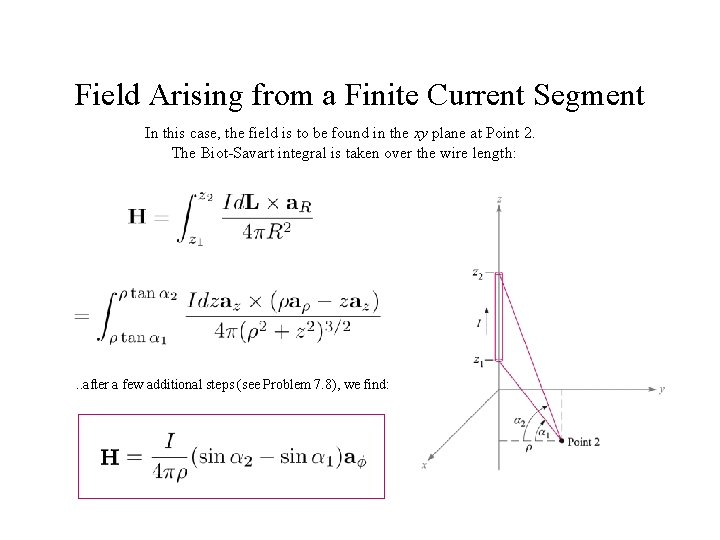Field Arising from a Finite Current Segment In this case, the field is to be found in the xy plane at Point 2. The Biot-Savart integral is taken over the wire length: . . after a few additional steps (see Problem 7. 8), we find:Another Example: Magnetic Field from a Current Loop Consider a circular current loop of radius a in the x-y plane, which carries steady current I. We wish to find the magnetic field strength anywhere on the z axis. We will use the Biot-Savart Law: where:Example: Continued Substituting the previous expressions, the Biot-Savart Law becomes: carry out the cross products to find: but we must include the angle dependence in the radial unit vector: with this substitution, the radial component will integrate to zero, meaning that all radial components will cancel on the z axis.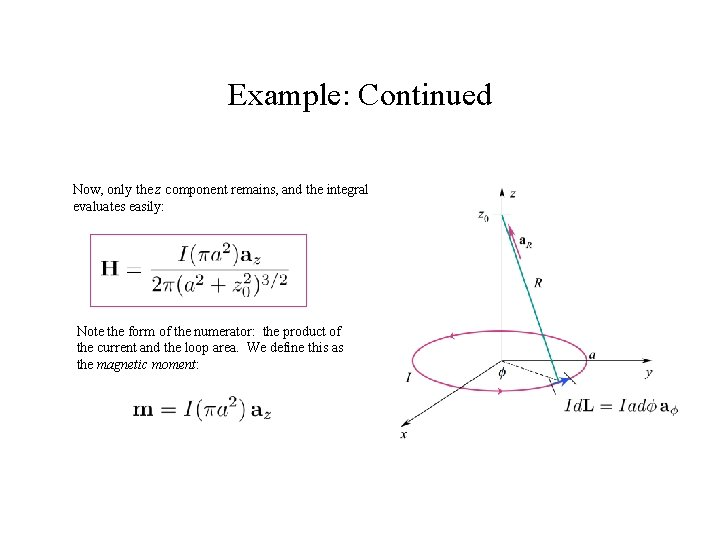Example: Continued Now, only the z component remains, and the integral evaluates easily: Note the form of the numerator: the product of the current and the loop area. We define this as the magnetic moment: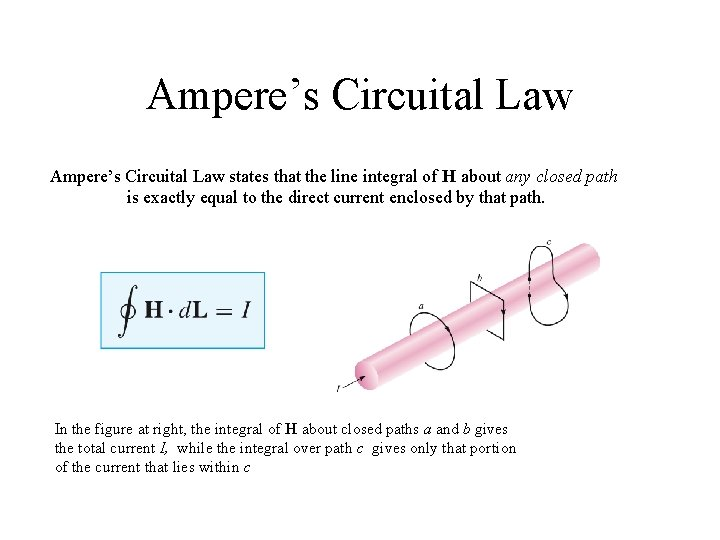Ampere’s Circuital Law states that the line integral of H about any closed path is exactly equal to the direct current enclosed by that path. In the figure at right, the integral of H about closed paths a and b gives the total current I, while the integral over path c gives only that portion of the current that lies within c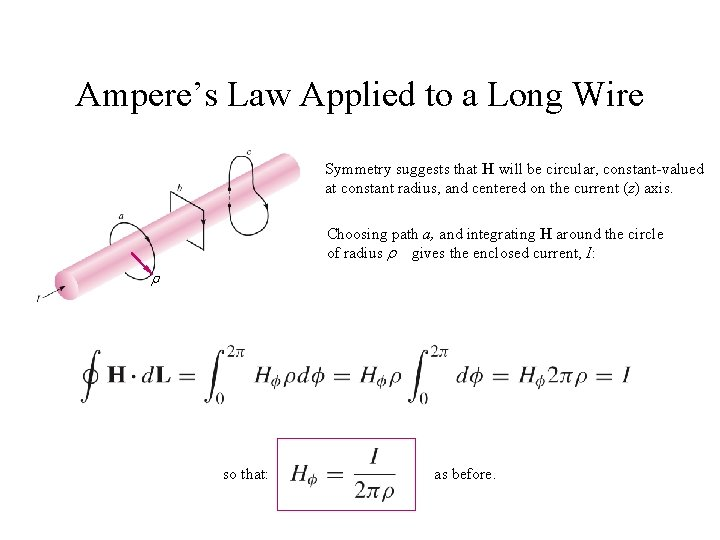Ampere’s Law Applied to a Long Wire Symmetry suggests that H will be circular, constant-valued at constant radius, and centered on the current (z) axis. Choosing path a, and integrating H around the circle of radius gives the enclosed current, I: so that: as before.Coaxial Transmission Line In the coax line, we have two concentric solid conductors that carry equal and opposite currents, I. The line is assumed to be infinitely long, and the circular symmetry suggests that H will be entirely - directed, and will vary only with radius . Our objective is to find the magnetic field for all values ofField Between Conductors The inner conductor can be thought of as made up of a bundle of filament currents, each of which produces the field of a long wire. Consider two such filaments, located at the same radius from the z axis, , but which lie at symmetric coordinates, and - Their field contributions superpose to give a net H component as shown. The same happens for every pair of symmetrically-located filaments, which taken as a whole, make up the entire center conductor. The field between conductors is thus found to be the same as that of filament conductor on the z axis that carries current, I. Specifically: a < < bField Within the Inner Conductor With current uniformly distributed inside the conductors, the H can be assumed circular everywhere. Inside the inner conductor, and at radius we again have: But now, the current enclosed is so that or finally: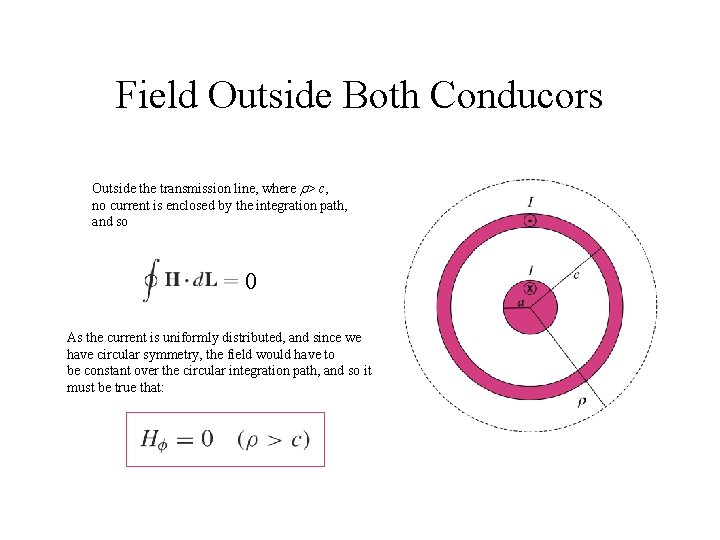Field Outside Both Conducors Outside the transmission line, where > c, no current is enclosed by the integration path, and so 0 As the current is uniformly distributed, and since we have circular symmetry, the field would have to be constant over the circular integration path, and so it must be true that:Field Inside the Outer Conductor Inside the outer conductor, the enclosed current consists of that within the inner conductor plus that portion of the outer conductor current existing at radii less than Ampere’s Circuital Law becomes . . and so finally:Magnetic Field Strength as a Function of Radius in the Coax Line Combining the previous results, and assigning dimensions as shown in the inset below, we find: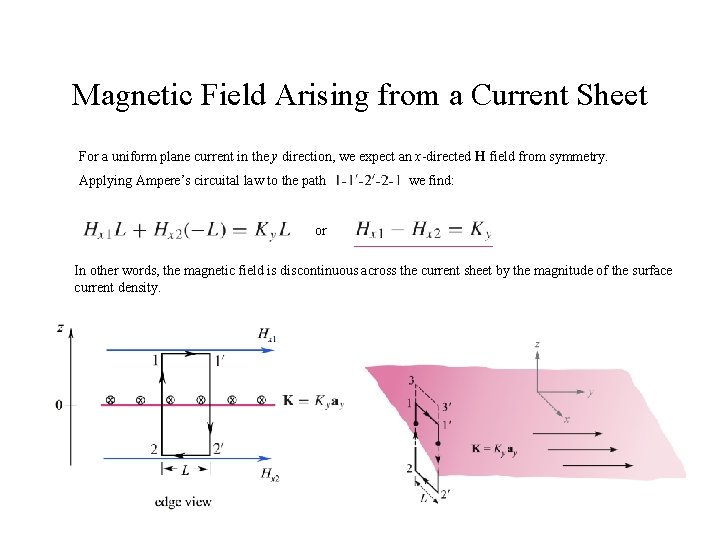Magnetic Field Arising from a Current Sheet For a uniform plane current in the y direction, we expect an x-directed H field from symmetry. Applying Ampere’s circuital law to the path we find: or In other words, the magnetic field is discontinuous across the current sheet by the magnitude of the surface current density.Magnetic Field Arising from a Current Sheet If instead, the upper path is elevated to the line between and , the same current is enclosed and we would have from which we conclude that so the field is constant in each region (above and below the current plane) By symmetry, the field above the sheet must be the same in magnitude as the field below the sheet. Therefore, we may state that and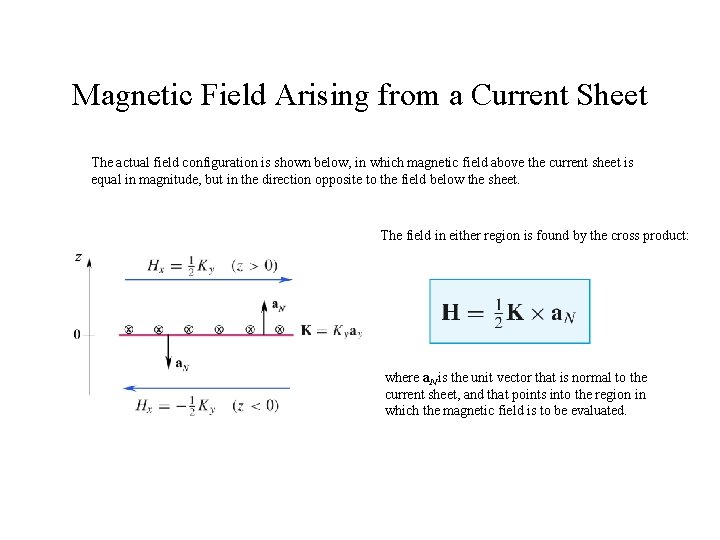Magnetic Field Arising from a Current Sheet The actual field configuration is shown below, in which magnetic field above the current sheet is equal in magnitude, but in the direction opposite to the field below the sheet. The field in either region is found by the cross product: where a. N is the unit vector that is normal to the current sheet, and that points into the region in which the magnetic field is to be evaluated.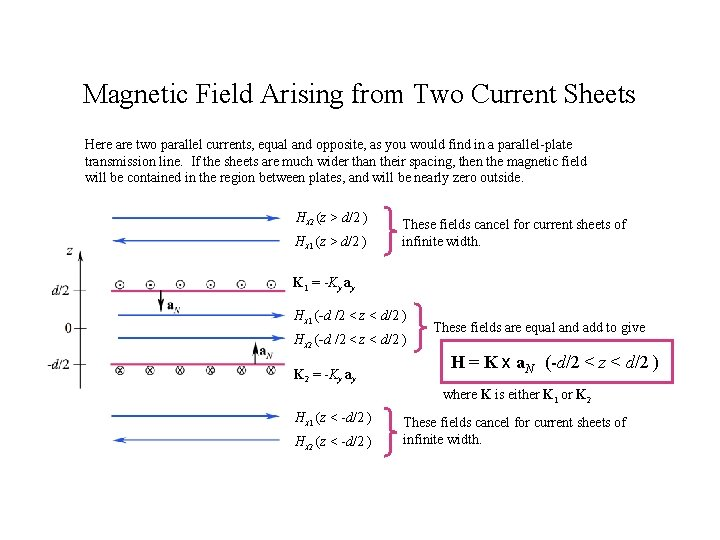Magnetic Field Arising from Two Current Sheets Here are two parallel currents, equal and opposite, as you would find in a parallel-plate transmission line. If the sheets are much wider than their spacing, then the magnetic field will be contained in the region between plates, and will be nearly zero outside. Hx 2 (z > d/2 ) Hx 1 (z > d/2 ) These fields cancel for current sheets of infinite width. K 1 = -Ky ay Hx 1 (-d /2 < z < d/2 ) Hx 2 (-d /2 < z < d/2 ) K 2 = -Ky ay These fields are equal and add to give H = K x a. N (-d/2 < z < d/2 ) where K is either K 1 or K 2 Hx 1 (z < -d/2 ) Hx 2 (z < -d/2 ) These fields cancel for current sheets of infinite width.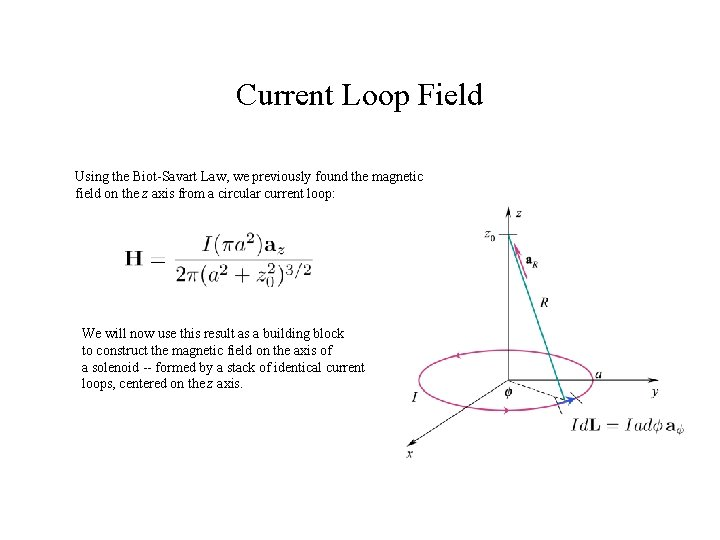Current Loop Field Using the Biot-Savart Law, we previously found the magnetic field on the z axis from a circular current loop: We will now use this result as a building block to construct the magnetic field on the axis of a solenoid -- formed by a stack of identical current loops, centered on the z axis.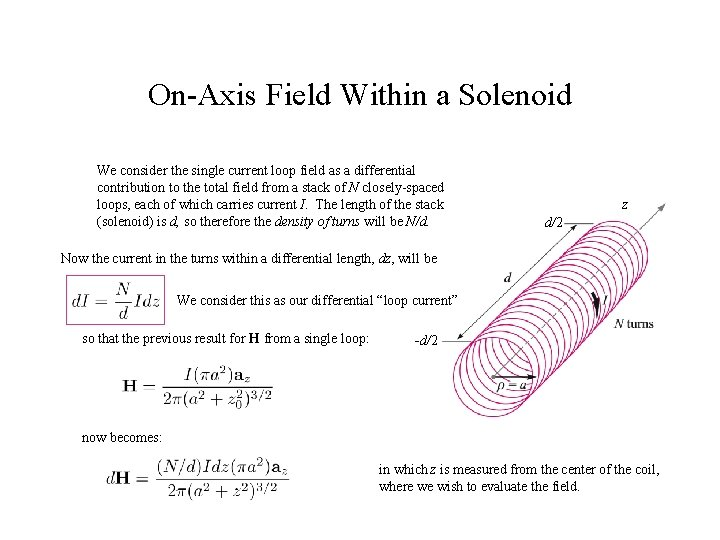On-Axis Field Within a Solenoid We consider the single current loop field as a differential contribution to the total field from a stack of N closely-spaced loops, each of which carries current I. The length of the stack (solenoid) is d, so therefore the density of turns will be N/d. z d/2 Now the current in the turns within a differential length, dz, will be We consider this as our differential “loop current” so that the previous result for H from a single loop: -d/2 now becomes: in which z is measured from the center of the coil, where we wish to evaluate the field.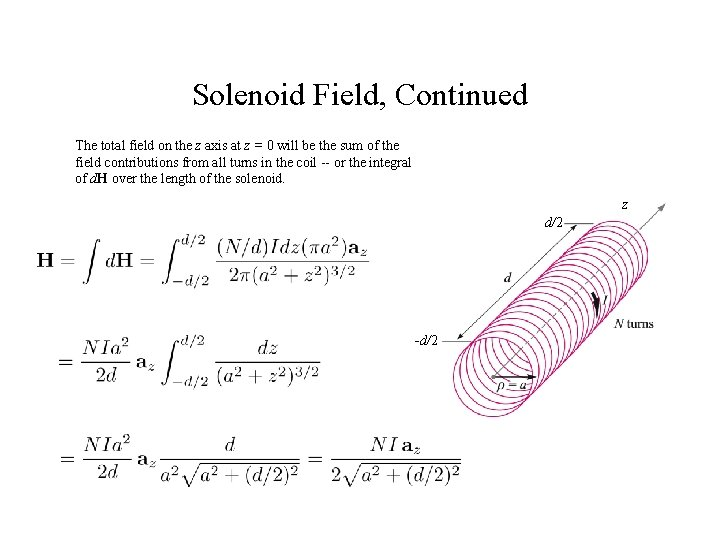Solenoid Field, Continued The total field on the z axis at z = 0 will be the sum of the field contributions from all turns in the coil -- or the integral of d. H over the length of the solenoid. z d/2 -d/2Approximation for Long Solenoids We now have the on-axis field at the solenoid midpoint (z = 0): z d/2 Note that for long solenoids, for which result simplifies to: , the -d/2 ( ) This result is valid at all on-axis positions deep within long coils -- at distances from each end of several radii.Another Interpretation: Continuous Surface Current The solenoid of our previous example was assumed to have many tightly-wound turns, with several existing within a differential length, dz. We could model such a current configuration as a continuous surface current of density K = Ka a A/m. d/2 Therefore: -d/2 In other words, the on-axis field magnitude near the center of a cylindrical current sheet, where current circulates around the z axis, and whose length is much greater than its radius, is just the surface current density.Solenoid Field -- Off-Axis To find the field within a solenoid, but off the z axis, we apply Ampere’s Circuital Law in the following way: The illustration below shows the solenoid cross-section, from a lengthwise cut through the z axis. Current in the windings flows in and out of the screen in the circular current path. Each turn carries current I. The magnetic field along the z axis is NI/d as we found earlier.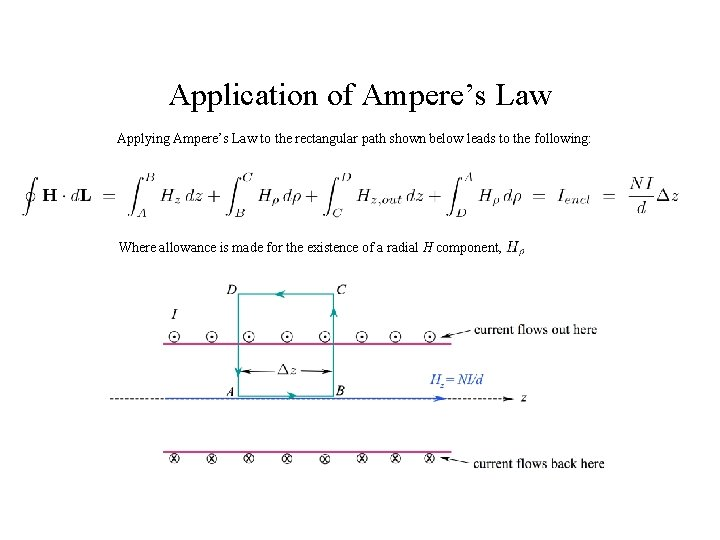Application of Ampere’s Law Applying Ampere’s Law to the rectangular path shown below leads to the following: Where allowance is made for the existence of a radial H component,Radial Path Segments The radial integrals will now cancel, because they are oppositely-directed, and because in the long coil, is not expected to differ between the two radial path segments.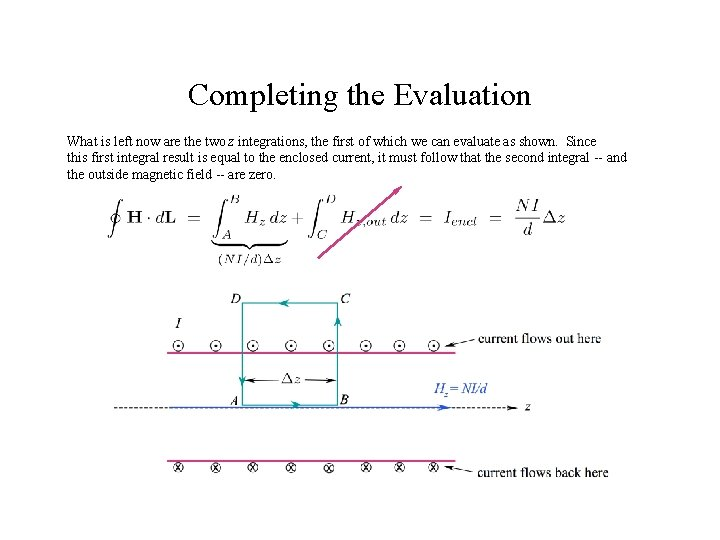Completing the Evaluation What is left now are the two z integrations, the first of which we can evaluate as shown. Since this first integral result is equal to the enclosed current, it must follow that the second integral -- and the outside magnetic field -- are zero.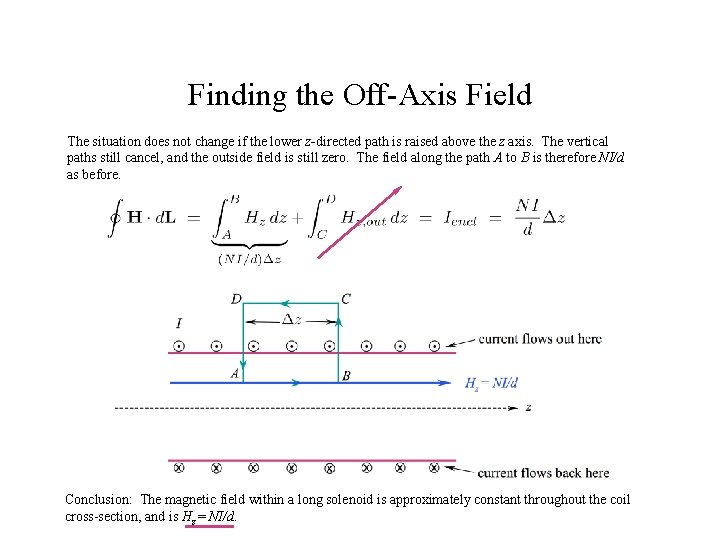Finding the Off-Axis Field The situation does not change if the lower z-directed path is raised above the z axis. The vertical paths still cancel, and the outside field is still zero. The field along the path A to B is therefore NI/d as before. Conclusion: The magnetic field within a long solenoid is approximately constant throughout the coil cross-section, and is Hz = NI/d.Toroid Magnetic Field A toroid is a doughnut-shaped set of windings around a core material. The cross-section could be circular (as shown here, with radius a) or any other shape. Below, a slice of the toroid is shown, with current emerging from the screen around the inner periphery (in the positive z direction). The windings are modeled as N individual current loops, each of which carries current I.Ampere’s Law as Applied to a Toroid Ampere’s Circuital Law can be applied to a toroid by taking a closed loop integral around the circular contour C at radius Magnetic field H is presumed to be circular, and a function of radius only at locations within the toroid that are not too close to the individual windings. Under this condition, we would assume: This approximation improves as the density of turns gets higher (using more turns with finer wire). Ampere’s Law now takes the form: so that…. Performing the same integrals over contours drawn in the regions or will lead to zero magnetic field there, because no current is enclosed in either case.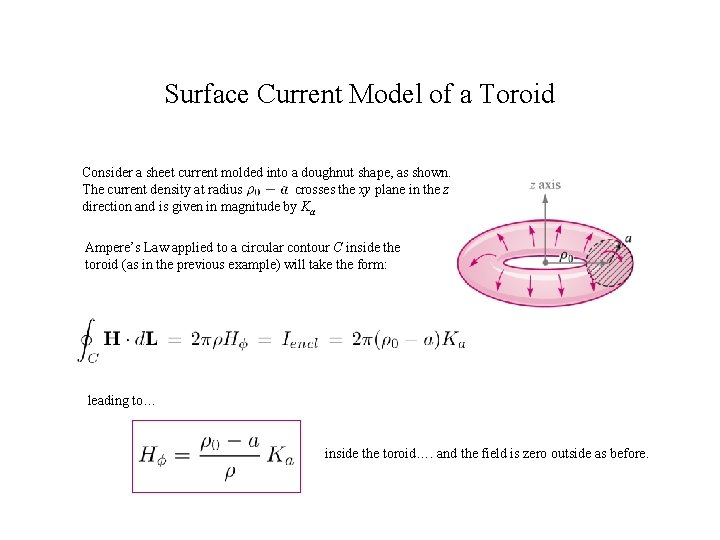Surface Current Model of a Toroid Consider a sheet current molded into a doughnut shape, as shown. The current density at radius crosses the xy plane in the z direction and is given in magnitude by Ka Ampere’s Law applied to a circular contour C inside the toroid (as in the previous example) will take the form: leading to… inside the toroid…. and the field is zero outside as before.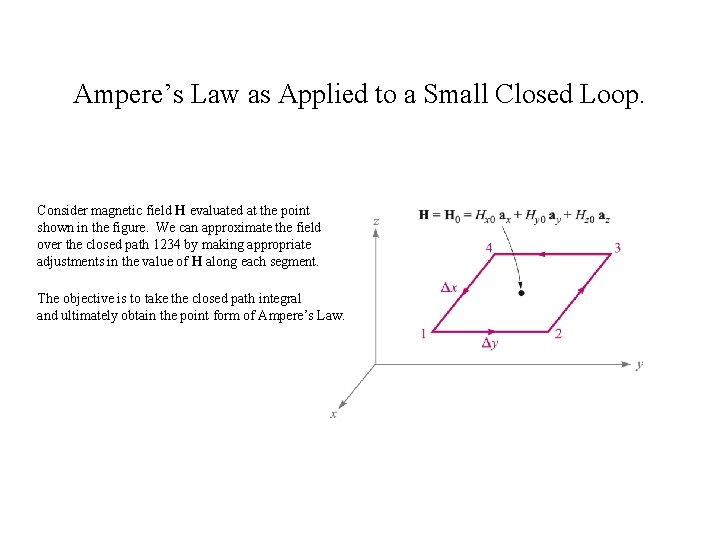Ampere’s Law as Applied to a Small Closed Loop. Consider magnetic field H evaluated at the point shown in the figure. We can approximate the field over the closed path 1234 by making appropriate adjustments in the value of H along each segment. The objective is to take the closed path integral and ultimately obtain the point form of Ampere’s Law.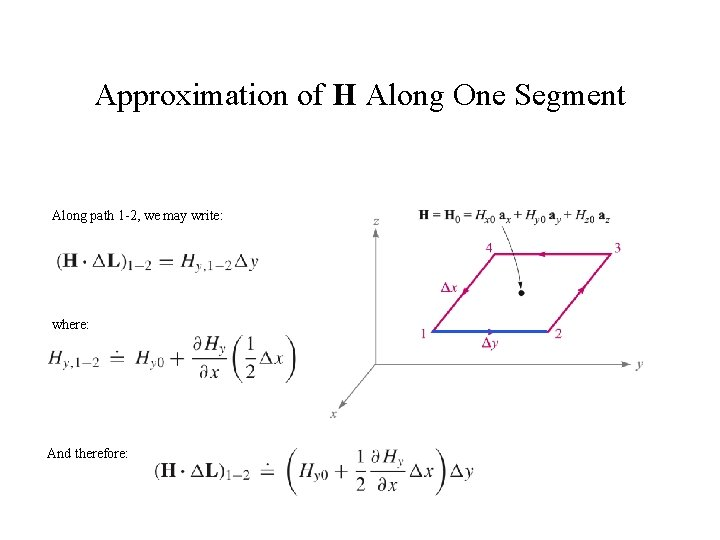Approximation of H Along One Segment Along path 1 -2, we may write: where: And therefore: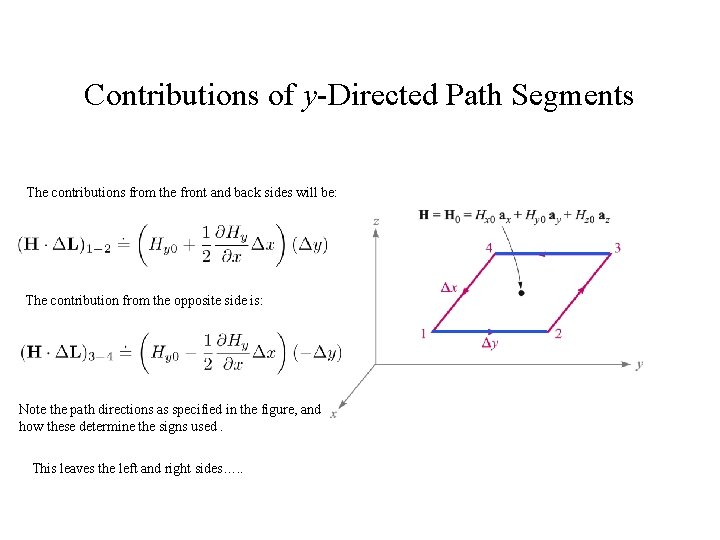Contributions of y-Directed Path Segments The contributions from the front and back sides will be: The contribution from the opposite side is: Note the path directions as specified in the figure, and how these determine the signs used. This leaves the left and right sides…. .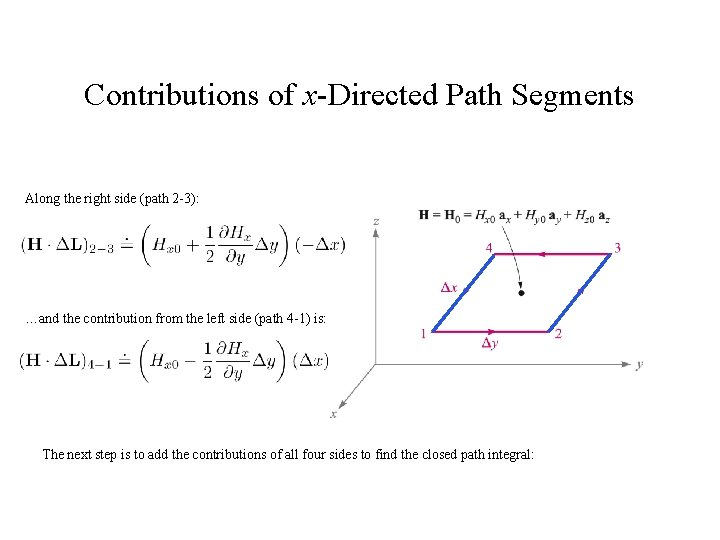Contributions of x-Directed Path Segments Along the right side (path 2 -3): …and the contribution from the left side (path 4 -1) is: The next step is to add the contributions of all four sides to find the closed path integral:Net Closed Path Integral The total integral will now be the sum: and using our previous results, the becomes: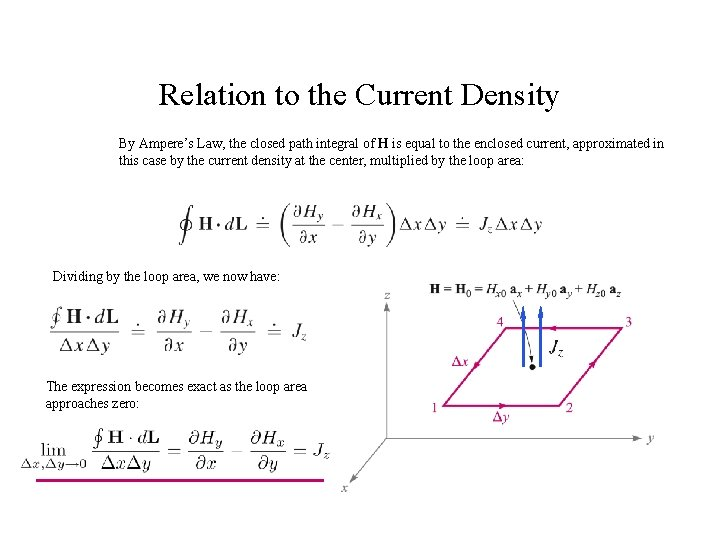Relation to the Current Density By Ampere’s Law, the closed path integral of H is equal to the enclosed current, approximated in this case by the current density at the center, multiplied by the loop area: Dividing by the loop area, we now have: The expression becomes exact as the loop area approaches zero: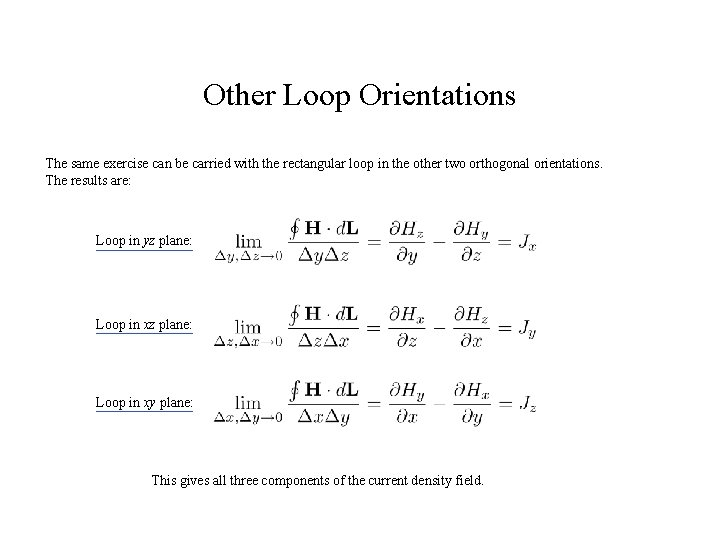Other Loop Orientations The same exercise can be carried with the rectangular loop in the other two orthogonal orientations. The results are: Loop in yz plane: Loop in xy plane: This gives all three components of the current density field.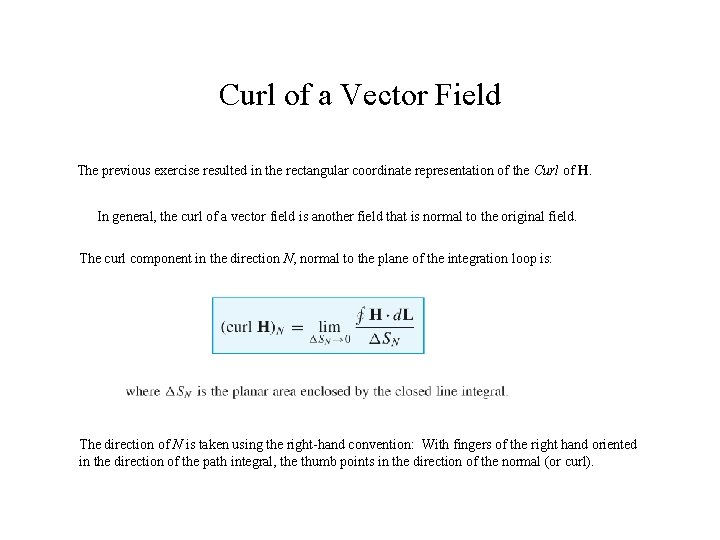Curl of a Vector Field The previous exercise resulted in the rectangular coordinate representation of the Curl of H. In general, the curl of a vector field is another field that is normal to the original field. The curl component in the direction N, normal to the plane of the integration loop is: The direction of N is taken using the right-hand convention: With fingers of the right hand oriented in the direction of the path integral, the thumb points in the direction of the normal (or curl).Curl in Rectangular Coordinates Assembling the results of the rectangular loop integration exercise, we find the vector field that comprises curl H: An easy way to calculate this is to evaluate the following determinant: which we see is equivalent to the cross product of the del operator with the field: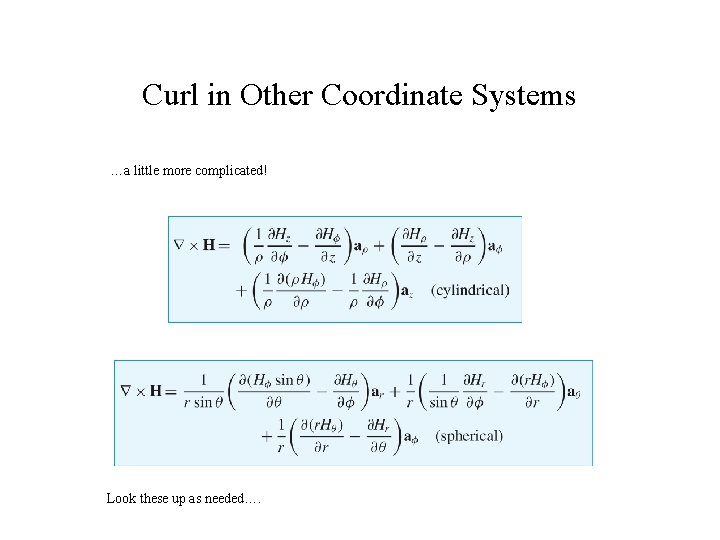Curl in Other Coordinate Systems …a little more complicated! Look these up as needed….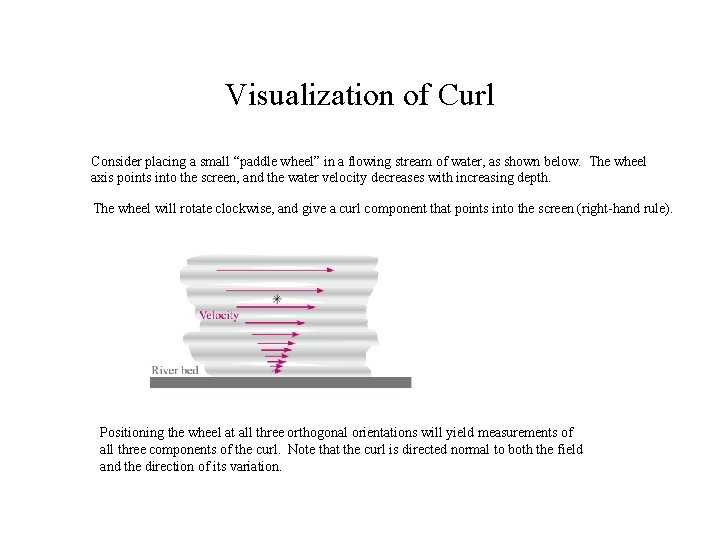Visualization of Curl Consider placing a small “paddle wheel” in a flowing stream of water, as shown below. The wheel axis points into the screen, and the water velocity decreases with increasing depth. The wheel will rotate clockwise, and give a curl component that points into the screen (right-hand rule). Positioning the wheel at all three orthogonal orientations will yield measurements of all three components of the curl. Note that the curl is directed normal to both the field and the direction of its variation.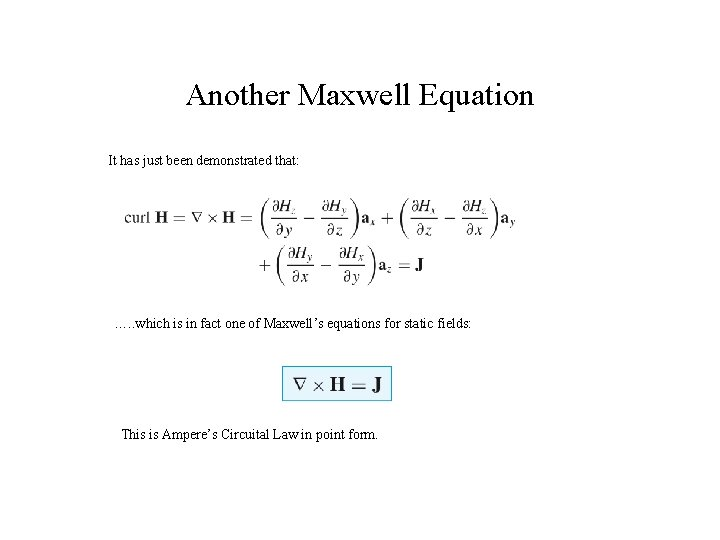Another Maxwell Equation It has just been demonstrated that: …. . which is in fact one of Maxwell’s equations for static fields: This is Ampere’s Circuital Law in point form.…. and Another Maxwell Equation We already know that for a static electric field: This means that: (applies to a static electric field) Recall the condition for a conservative field: that is, its closed path integral is zero everywhere. Therefore, a field is conservative if it has zero curl at all points over which the field is defined.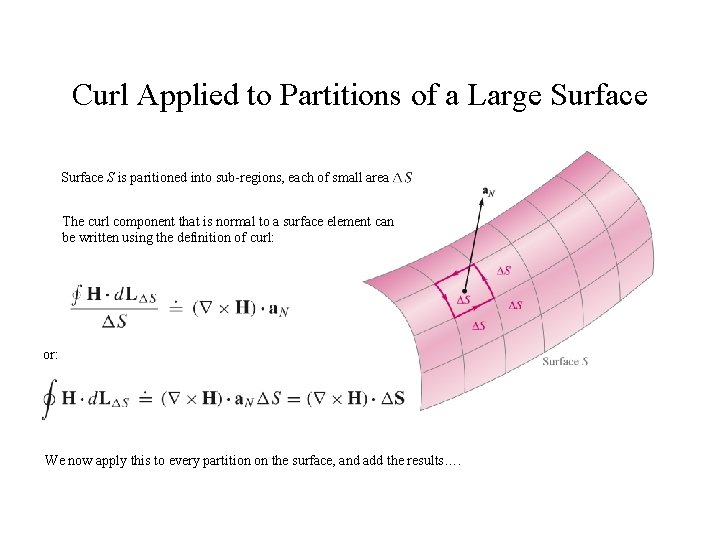Curl Applied to Partitions of a Large Surface S is paritioned into sub-regions, each of small area The curl component that is normal to a surface element can be written using the definition of curl: or: We now apply this to every partition on the surface, and add the results….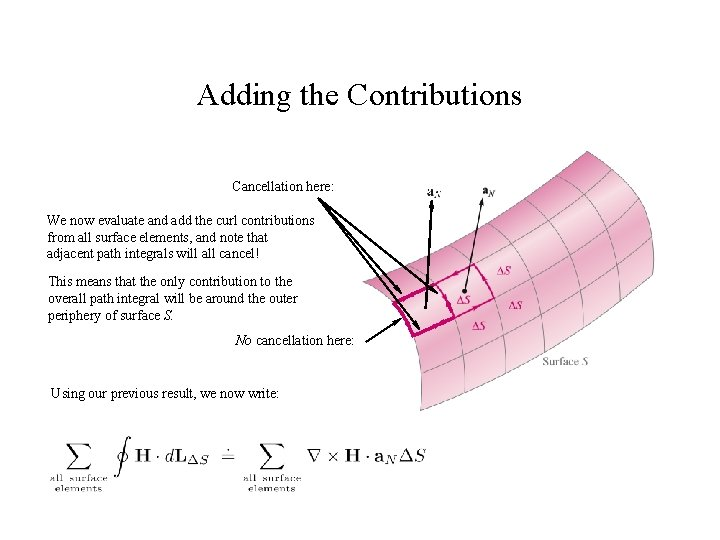Adding the Contributions Cancellation here: We now evaluate and add the curl contributions from all surface elements, and note that adjacent path integrals will all cancel! This means that the only contribution to the overall path integral will be around the outer periphery of surface S. No cancellation here: Using our previous result, we now write: .Stokes’ Theorem We now take our previous result, and take the limit as In the limit, this side becomes the path integral of H over the outer perimeter because all interior paths cancel In the limit, this side becomes the integral of the curl of H over surface S . The result is Stokes’ Theorem This is a valuable tool to have at our disposal, because it gives us two ways to evaluate the same thing!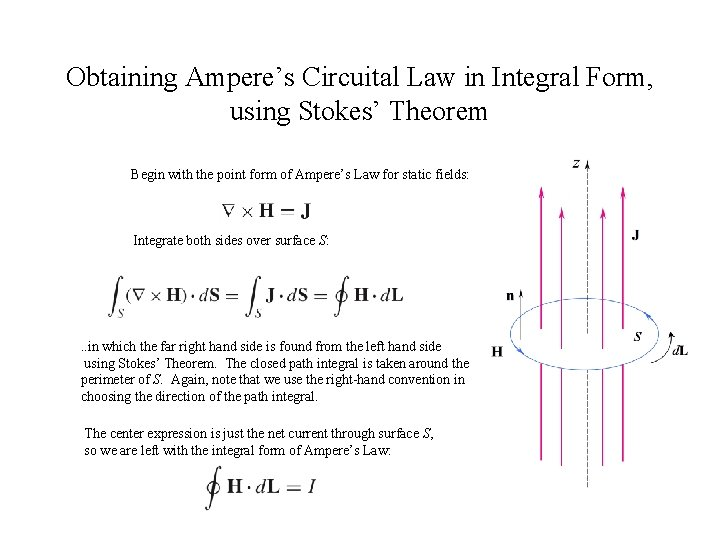Obtaining Ampere’s Circuital Law in Integral Form, using Stokes’ Theorem Begin with the point form of Ampere’s Law for static fields: Integrate both sides over surface S: . . in which the far right hand side is found from the left hand side using Stokes’ Theorem. The closed path integral is taken around the perimeter of S. Again, note that we use the right-hand convention in choosing the direction of the path integral. The center expression is just the net current through surface S, so we are left with the integral form of Ampere’s Law: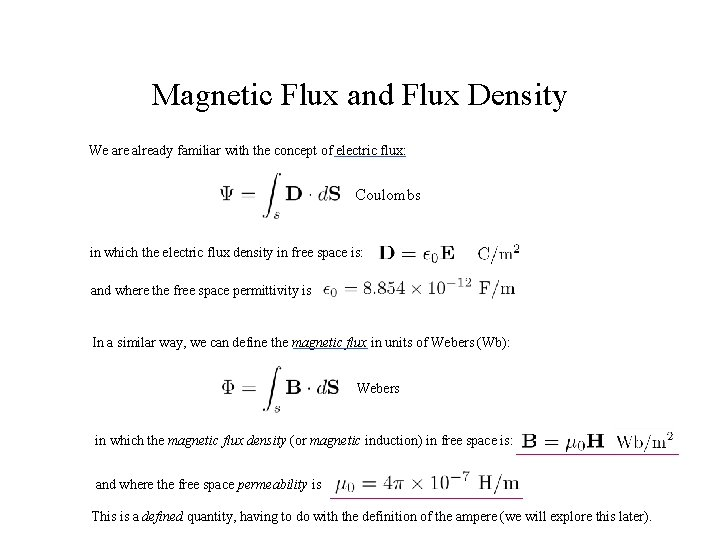Magnetic Flux and Flux Density We are already familiar with the concept of electric flux: Coulombs in which the electric flux density in free space is: and where the free space permittivity is In a similar way, we can define the magnetic flux in units of Webers (Wb): Webers in which the magnetic flux density (or magnetic induction) in free space is: and where the free space permeability is This is a defined quantity, having to do with the definition of the ampere (we will explore this later).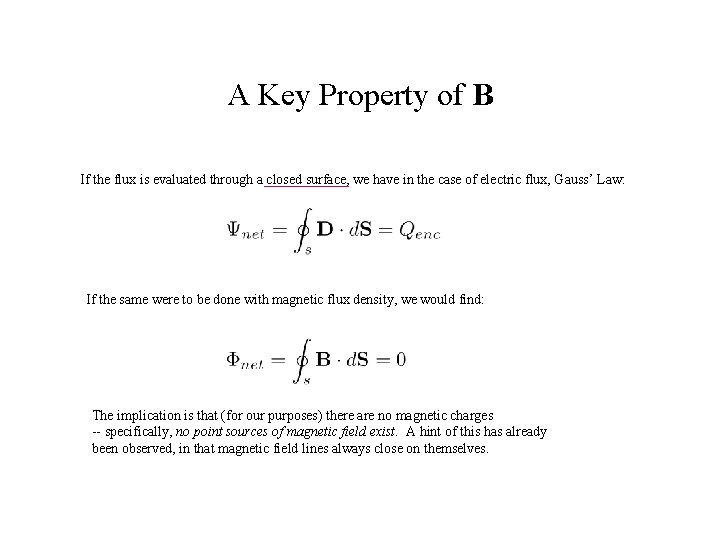A Key Property of B If the flux is evaluated through a closed surface, we have in the case of electric flux, Gauss’ Law: If the same were to be done with magnetic flux density, we would find: The implication is that (for our purposes) there are no magnetic charges -- specifically, no point sources of magnetic field exist. A hint of this has already been observed, in that magnetic field lines always close on themselves.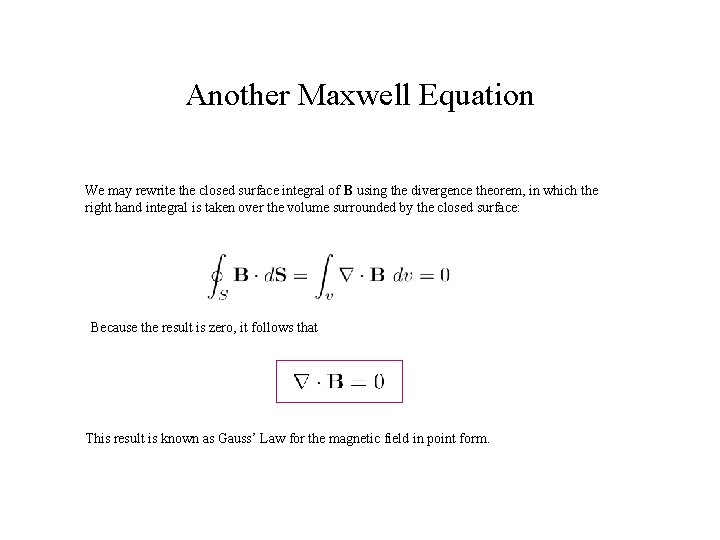Another Maxwell Equation We may rewrite the closed surface integral of B using the divergence theorem, in which the right hand integral is taken over the volume surrounded by the closed surface: Because the result is zero, it follows that This result is known as Gauss’ Law for the magnetic field in point form.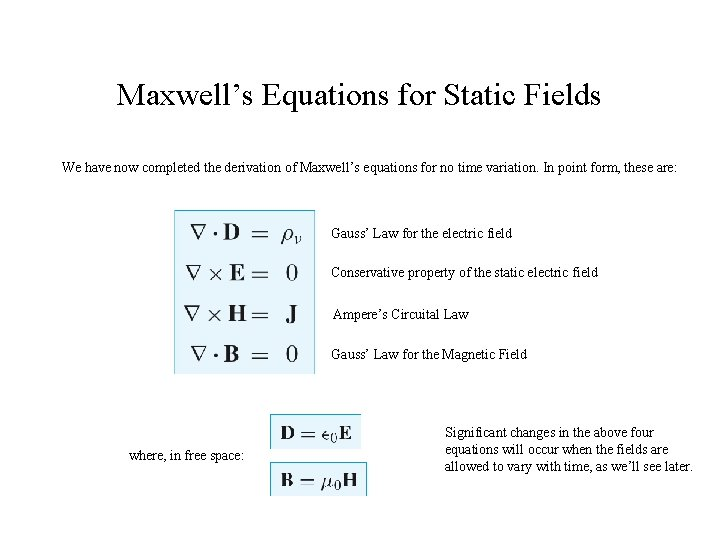Maxwell’s Equations for Static Fields We have now completed the derivation of Maxwell’s equations for no time variation. In point form, these are: Gauss’ Law for the electric field Conservative property of the static electric field Ampere’s Circuital Law Gauss’ Law for the Magnetic Field where, in free space: Significant changes in the above four equations will occur when the fields are allowed to vary with time, as we’ll see later.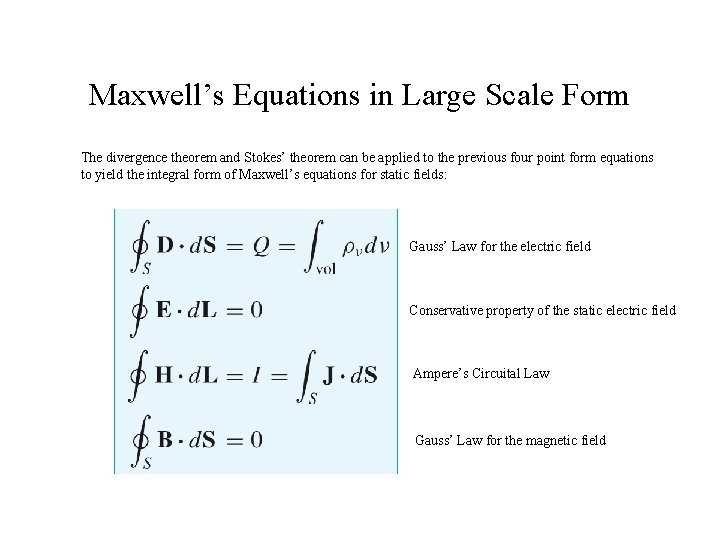Maxwell’s Equations in Large Scale Form The divergence theorem and Stokes’ theorem can be applied to the previous four point form equations to yield the integral form of Maxwell’s equations for static fields: Gauss’ Law for the electric field Conservative property of the static electric field Ampere’s Circuital Law Gauss’ Law for the magnetic field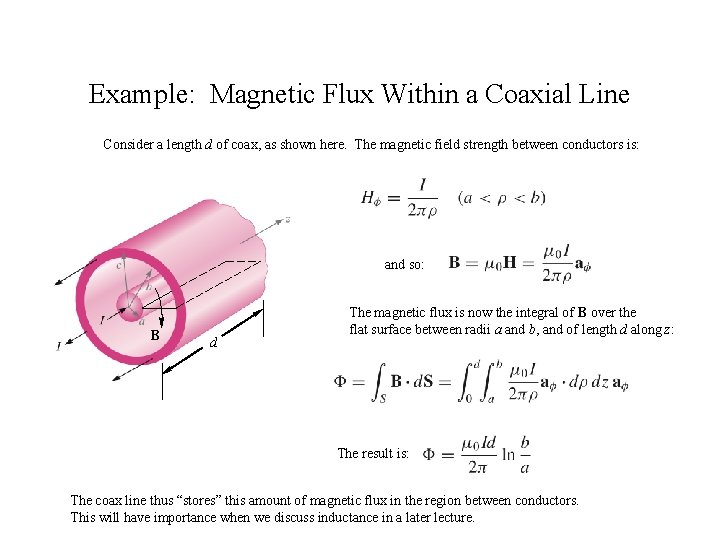Example: Magnetic Flux Within a Coaxial Line Consider a length d of coax, as shown here. The magnetic field strength between conductors is: and so: B d The magnetic flux is now the integral of B over the flat surface between radii a and b, and of length d along z: The result is: The coax line thus “stores” this amount of magnetic flux in the region between conductors. This will have importance when we discuss inductance in a later lecture.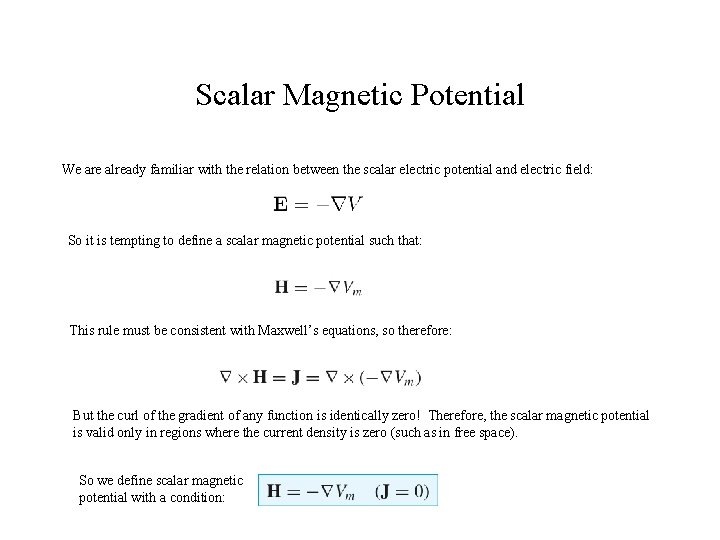Scalar Magnetic Potential We are already familiar with the relation between the scalar electric potential and electric field: So it is tempting to define a scalar magnetic potential such that: This rule must be consistent with Maxwell’s equations, so therefore: But the curl of the gradient of any function is identically zero! Therefore, the scalar magnetic potential is valid only in regions where the current density is zero (such as in free space). So we define scalar magnetic potential with a condition: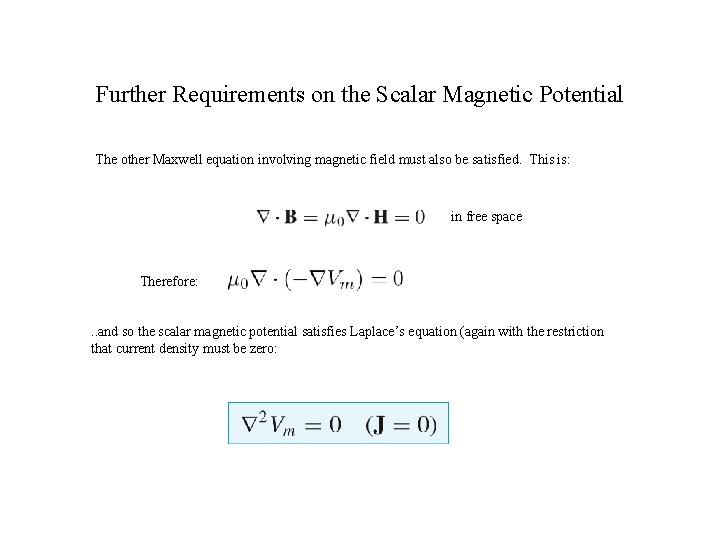Further Requirements on the Scalar Magnetic Potential The other Maxwell equation involving magnetic field must also be satisfied. This is: in free space Therefore: . . and so the scalar magnetic potential satisfies Laplace’s equation (again with the restriction that current density must be zero: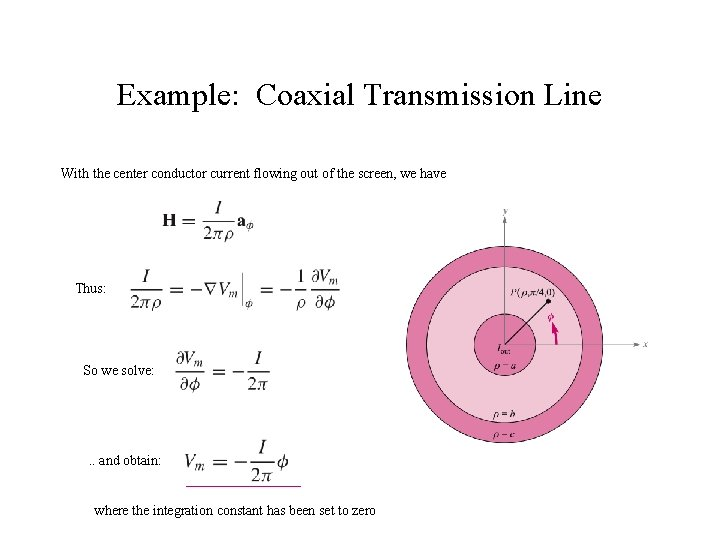Example: Coaxial Transmission Line With the center conductor current flowing out of the screen, we have Thus: So we solve: . . and obtain: where the integration constant has been set to zeroAmbiguities in the Scalar Potential The scalar potential is now: where the potential is zero at At point P ( ) the potential is But wait! As increases to we have returned to the same physical location, and the potential has a new value of -I. In general, the potential at P will be multivalued, and will acquire a new value after each full rotation in the xy plane: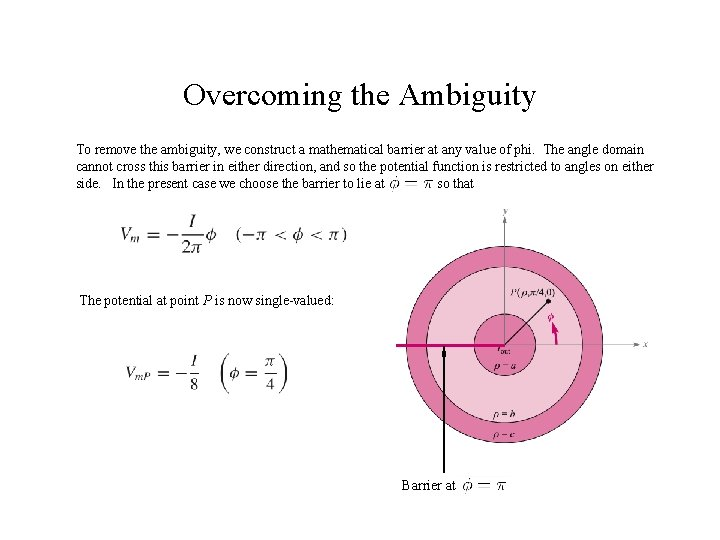Overcoming the Ambiguity To remove the ambiguity, we construct a mathematical barrier at any value of phi. The angle domain cannot cross this barrier in either direction, and so the potential function is restricted to angles on either side. In the present case we choose the barrier to lie at so that The potential at point P is now single-valued: Barrier atVector Magnetic Potential We make use of the Maxwell equation: . . and the fact that the divergence of the curl of any vector field is identically zero (show this!) This leads to the definition of the magnetic vector potential, A: Thus: and Ampere’s Law becomes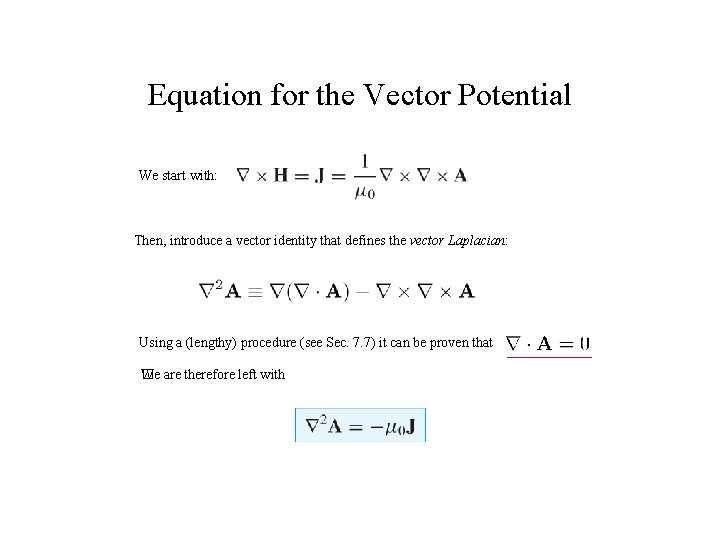Equation for the Vector Potential We start with: Then, introduce a vector identity that defines the vector Laplacian: Using a (lengthy) procedure (see Sec. 7. 7) it can be proven that We are therefore left with �The Direction of A We now have In rectangular coordinates: (not so simple in the other coordinate systems) The equation separates to give: This indicates that the direction of A will be the same as that of the current to which it is associated. The vector field, A, existing in all space, is sometimes described as being a “fuzzy image” of its generating current.Expressions for Potential Consider a differential elements, shown here. On the left is a point charge represented by a differential length of line charge. On the right is a differential current element. The setups for obtaining potential are identical between the two cases. Line Charge Scalar Electrostatic Potential Line Current Vector Magnetic PotentialGeneral Expressions for Vector Potential For large scale charge or current distributions, we would sum the differential contributions by integrating over the charge or current, thus: and The closed path integral is taken because the current must close on itself to form a complete circuit. For surface or volume current distributions, we would have, respectively: or in the same manner that we used for scalar electric potential.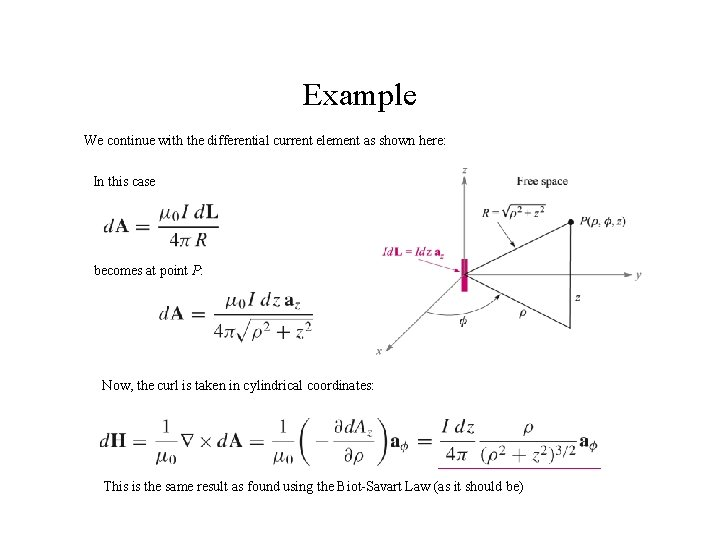Example We continue with the differential current element as shown here: In this case becomes at point P: Now, the curl is taken in cylindrical coordinates: This is the same result as found using the Biot-Savart Law (as it should be)